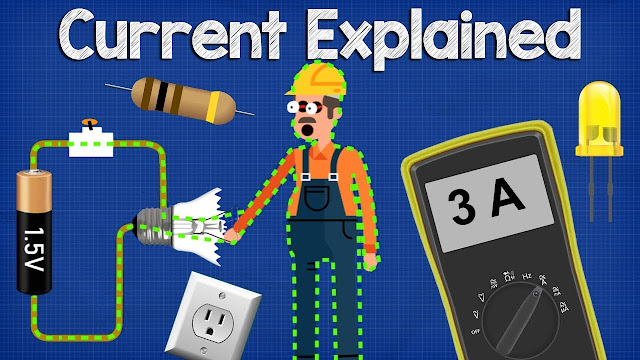What is CURRENT– electric current explained, electricity basics

# What is CURRENT– electric current explained, electricity basicsWhat is CURRENT– electric current explained, electricity basics

An electric current is the rate of flow of electric charge past a point :622 or region. :614 An electric current is said to exist when there is a net flow of electric charge through a region. :832 Electric charge is carried by charged particles, so an electric current is a flow of charged particles.
The moving particles are called charge carriers, and in different conductors may be different types of particle. In electric circuits the charge carriers are often electrons moving through a wire. In an electrolyte the charge carriers are ions, and in an ionized gas (plasma) are ions and electrons.

The SI unit of electric current is the ampere, which is the flow of electric charge across a surface at the rate of one coulomb per second. The ampere (symbol: A) is an SI base unit :15 Electric current is measured using a device called an ammeter. :788

Electric currents cause Joule heating, which creates light in incandescent light bulbs. They also create magnetic fields, which are used in motors, generators, inductors, and transformers.

What is electric current, in this video we learn what is electric current covering amps, coulombs, voltage, parallel and series circuits, multimeters, resistors, fuses and circuit breakers to learn how electricity works
Related topics:

Continue Reading about the "What is CURRENT– electric current explained, electricity basics" on the next page below